The work presented here has been done by Michele Caldoro, Marie Farge and Kai Schneider

## LABORATORY EXPERIMENT

This experiment has been realised by Jori E. Ruppert-Felsot, Emilie Regul, Eran Sharon et Harry L. Swinney at the Center for Nonlinear Dynamics, University of Texas using a rotating water tank with a forcing at the bottom. the vorticity is measured using particle image velocimetry (PIV) at a resolution N=1282.

### WAVELETS EXTRACTION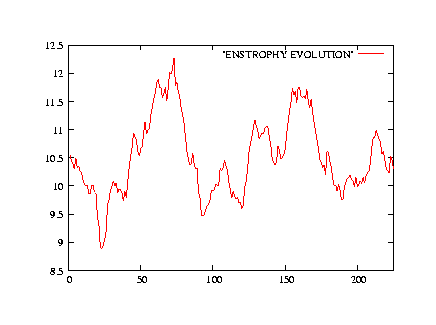Time evolution of the total enstrophy ZTime evolution of the maximal, minimum and mean vorticity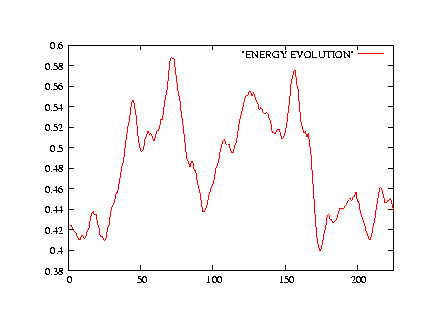Time evolution of the total energy E

We split the total flow into coherent and incoherent flows using the CVS filtering method with the Coifman 12 wavelet.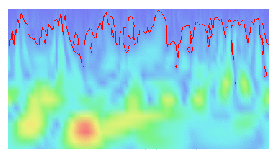Cut of the wavelet space with the treshold (red) separating coherent and incoherent wavelet modes

The percentage of retained coefficient is 4.9% N.
The percentage of retained enstrophy is 86.5% Z.

Total vorticity (min=-15.30 / max=33.53)

Coherent vorticity (min=-15.56 / max=34.09) and
incoherent vorticity (min=-3.25 / max=2.53)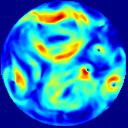Total velocity modulus (min=0 / max=2.20)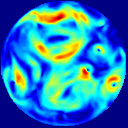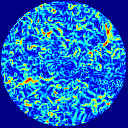Coherent velocity modulus (min=0 / max=2.22) and
incoherent velocity modulus (min=0 / max=0.09)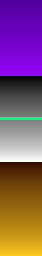Total streamfunction (min=-1.26 / max=1.28)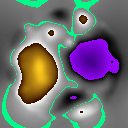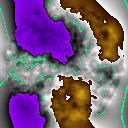Coherent streamfunction (min=-1.26 / max=1.28) and
incoherent streamfunction (min=-0.02 / max=0.02)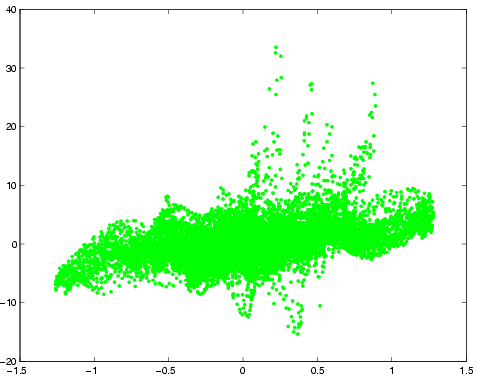Vorticity versus streamfunction for the total flow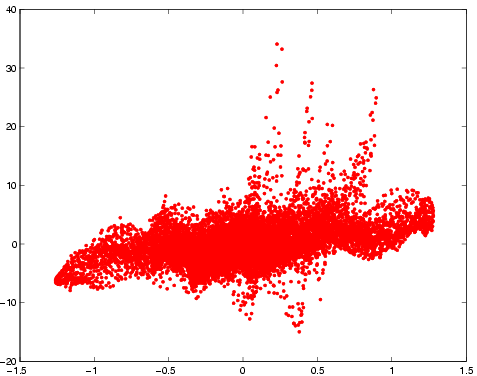Vorticity versus streamfunction for the coherent flowVorticity versus streamfunction for the incoherent flow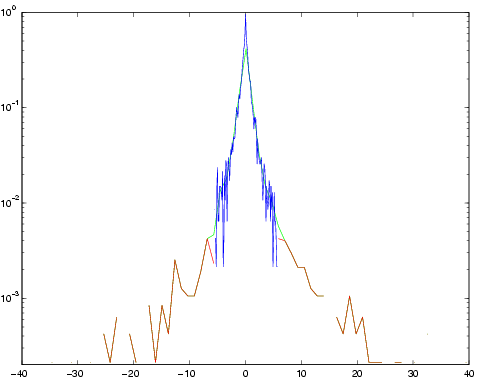Probability density function (PDF) of the wavelet coefficients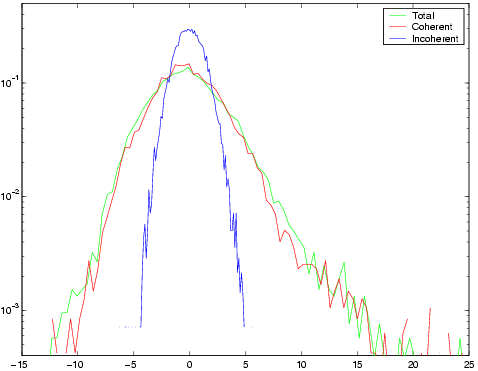Probability density function (PDF) of vorticityEnstrophy spectrum in lin-lin coordinates
(total: green, coherent: red, incoherent: blue)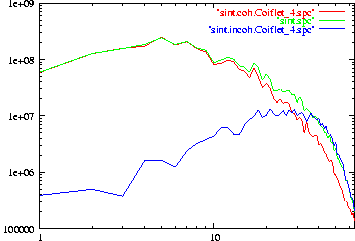Enstrophy spectra in log-log coordinates
(total: green, coherent: red, incoherent: blue)

### WAVELET PAKETS EXTRACTIONTotal vorticity (min=-15.30 / max=33.53)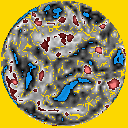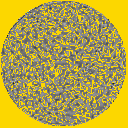Coherent vorticity (min=-16.10 / max=33.12) and
incoherent vorticity (min=-5.28 / max=4.36)Total velocity modulus (min=0 / max=2.20)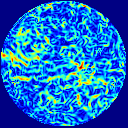Coherent velocity modulus (min=0 / max=2.21) and
incoherent velocity modulus (min=0 / max=0.13)Total streamfunction (min=-1.26 / max=1.28)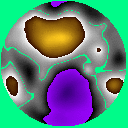Coherent streamfunction (min=-1.28 / max=1.30) and
incoherent streamfunction (min=-0.06 / max=0.05)Vorticity versus streamfunction for the total flow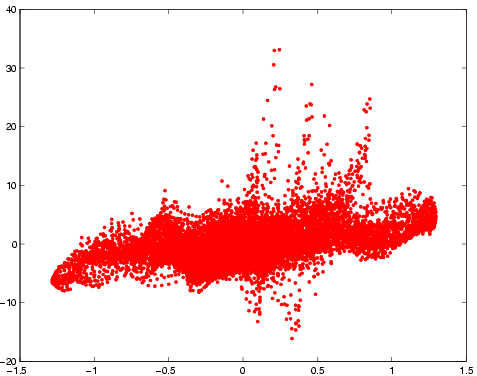Vorticity versus streamfunction for the coherent flow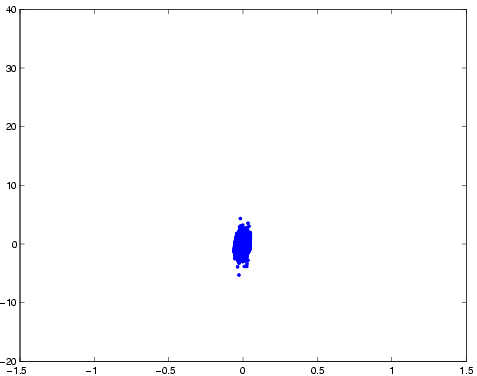Vorticity versus streamfunction for the incoherent flow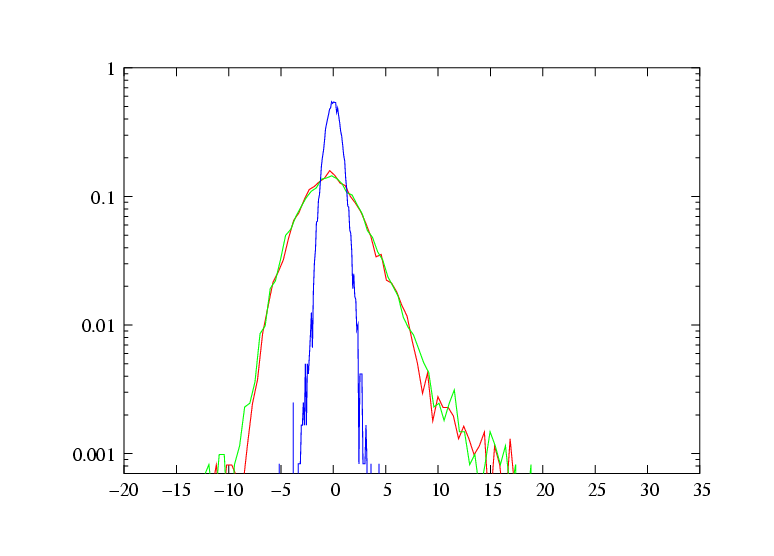Probability density function (PDF) of vorticityEnstrophy spectrum in lin-lin coordinates
(total: green, coherent: red, incoherent: blue)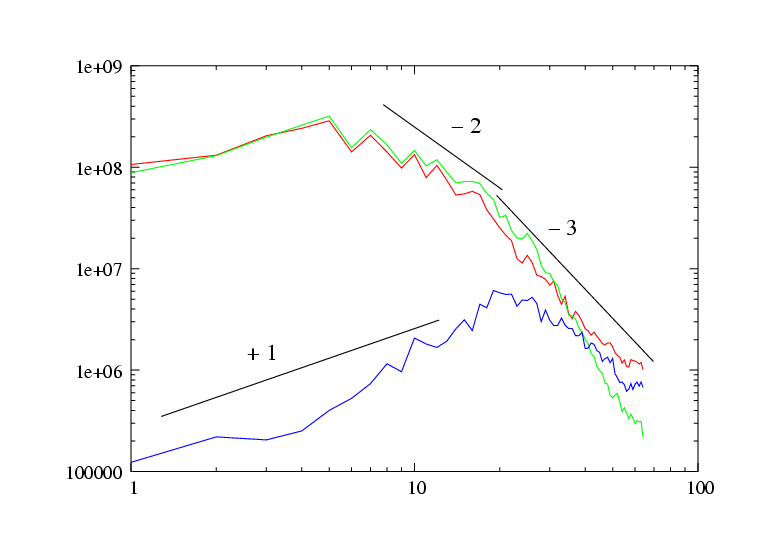Enstrophy spectra in log-log coordinates
(total: green, coherent: red, incoherent: blue)

## NUMERICAL SIMULATION

### 2D INCOMPRESSIBLE FLOW: DNS WITH NAVIER-STOKES EQUATIONS

This experiment has been performed by Kai Schneider, CMI, Universit� de Provence (Marseille), using a pseudo-spectral code integrating the two-dimensional Navier-Stokes equations without forcing. No-slip boundary conditions have been imposed in the cylindrical domain using volume penalisation.
The resolution is N=10242.Time evolution of the total enstophy z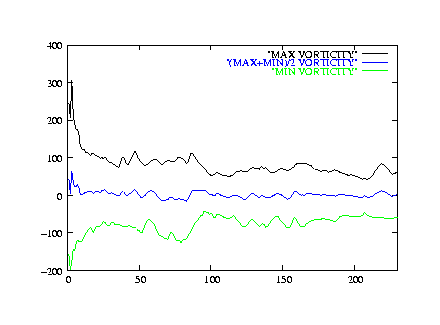Time evolution of the maximal, minimal and mean vorticity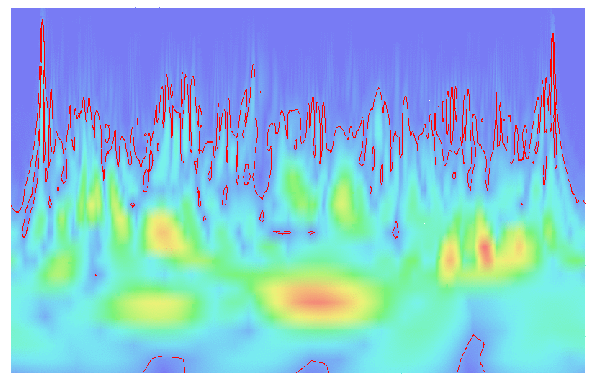Cut of the wavelet space with the treshold (red) separating coherent and incoherent wavelet modes

The percentage of retained coefficient is 6.8% N.
The percentage of retained enstrophy is 98.9% Z.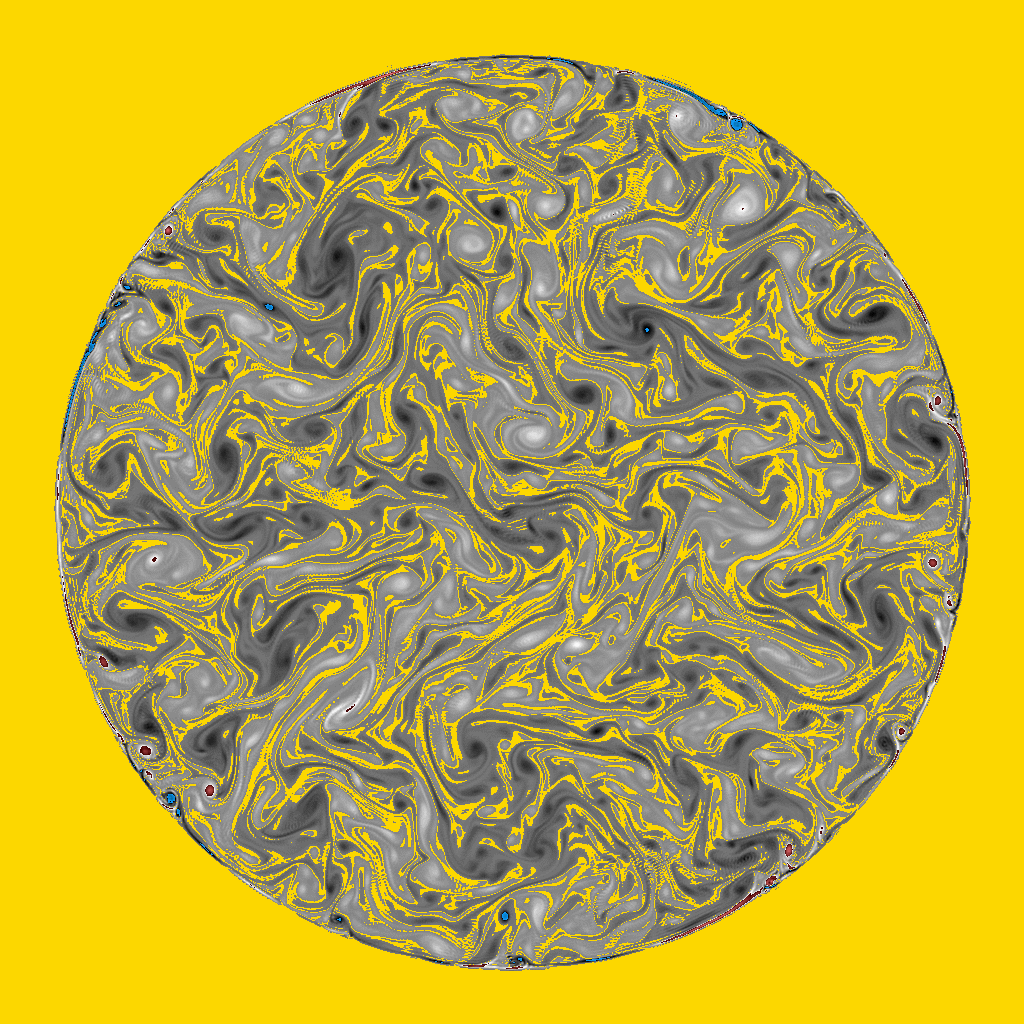Total vorticity (min=-123.63 / max=124.44)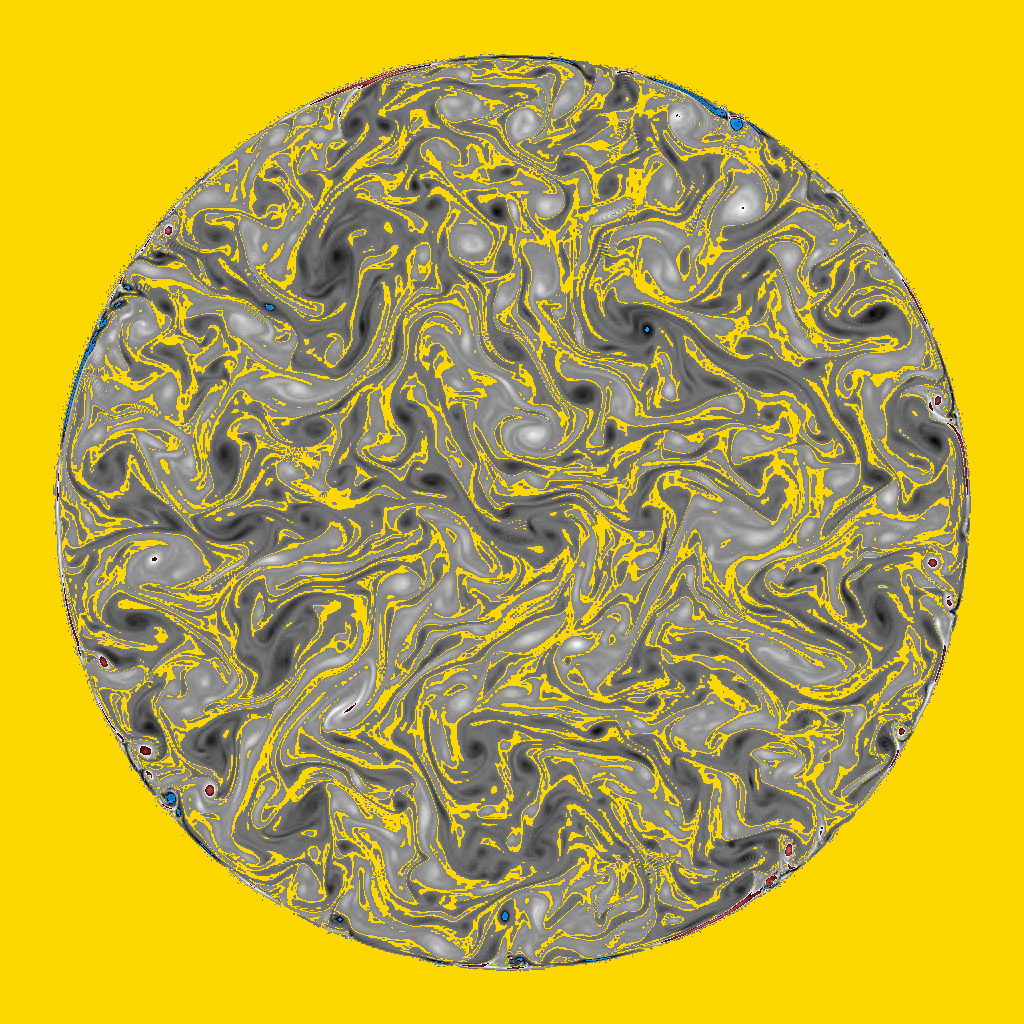Coherent vorticity (min=-123 / max=122.9) and
incoherent vorticity (min=-6.78 / max=7.09)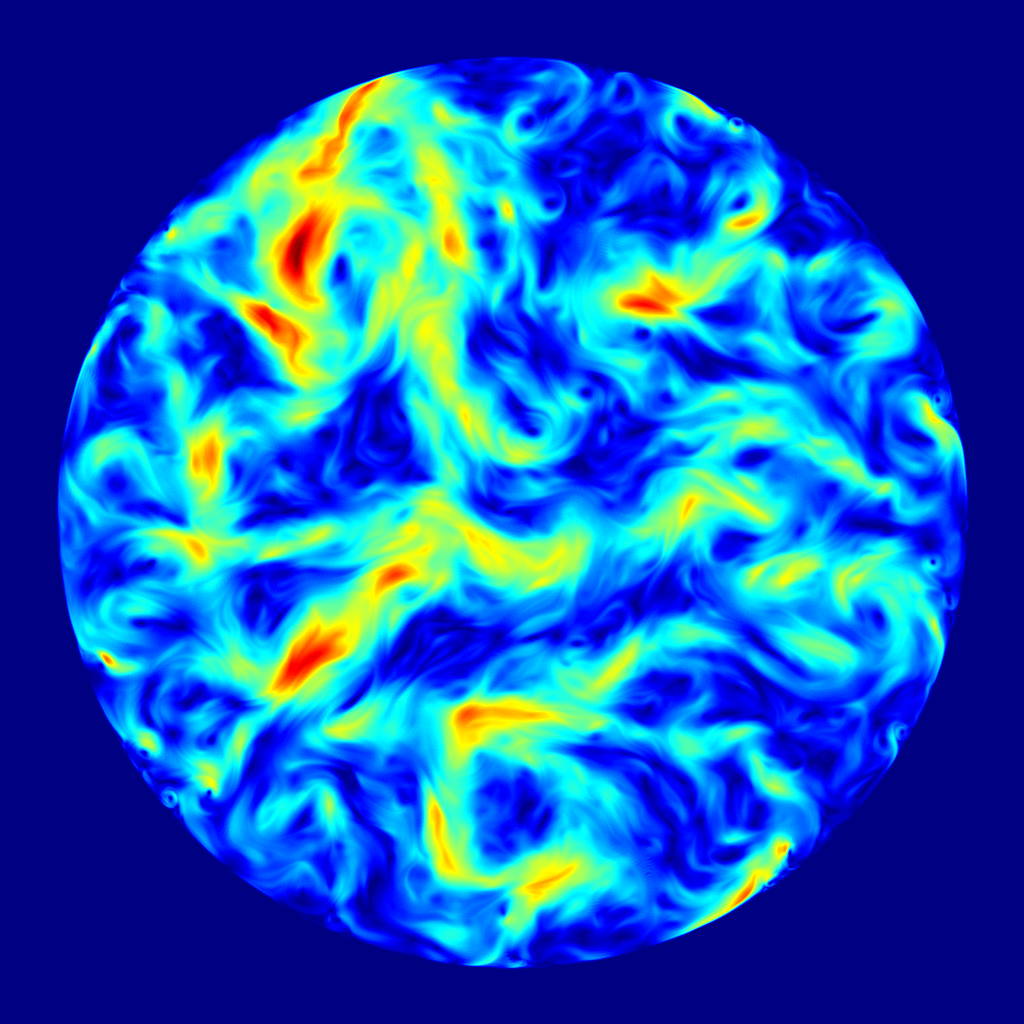Total velocity modulus (min=0 / max=2.67)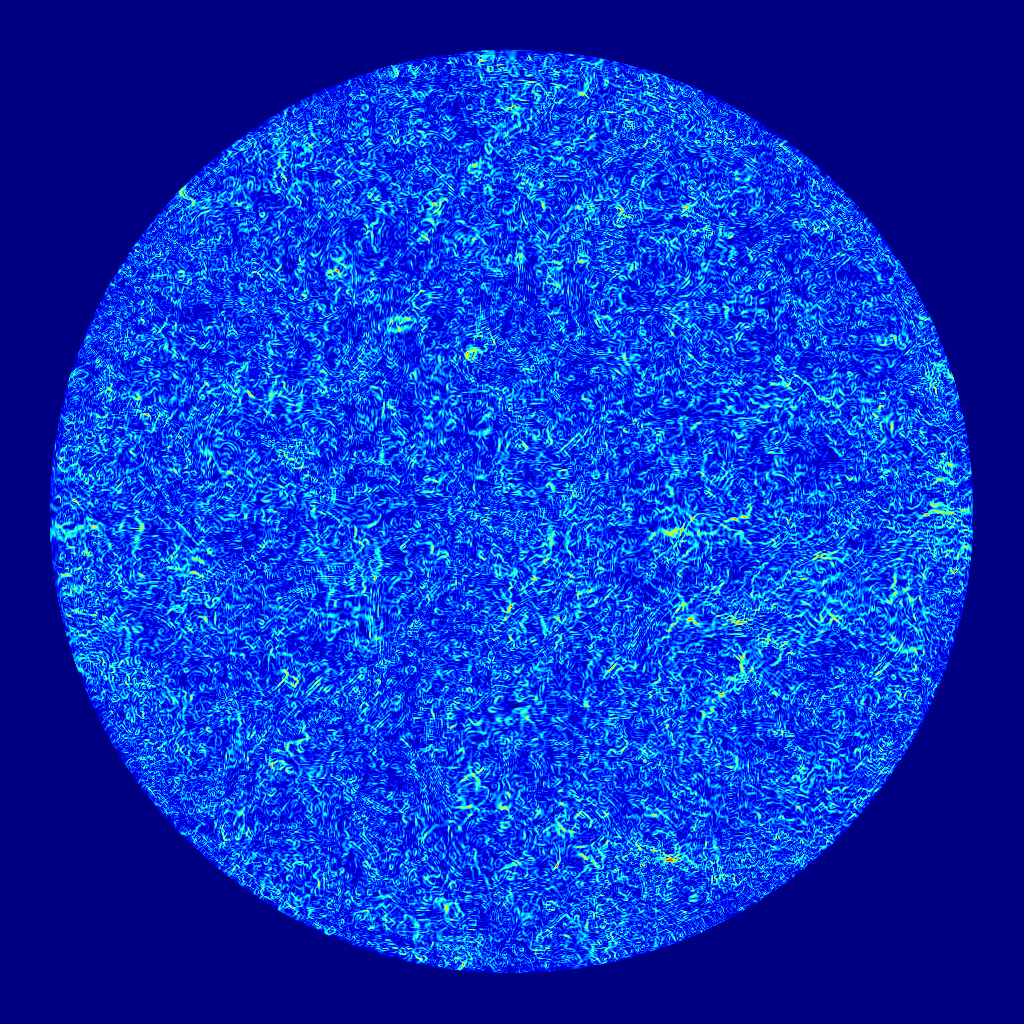Figure: Coherent velocity modulus (min=0 / max=2.67) and
incoherent velocity modulus (min=0 / max=0.03)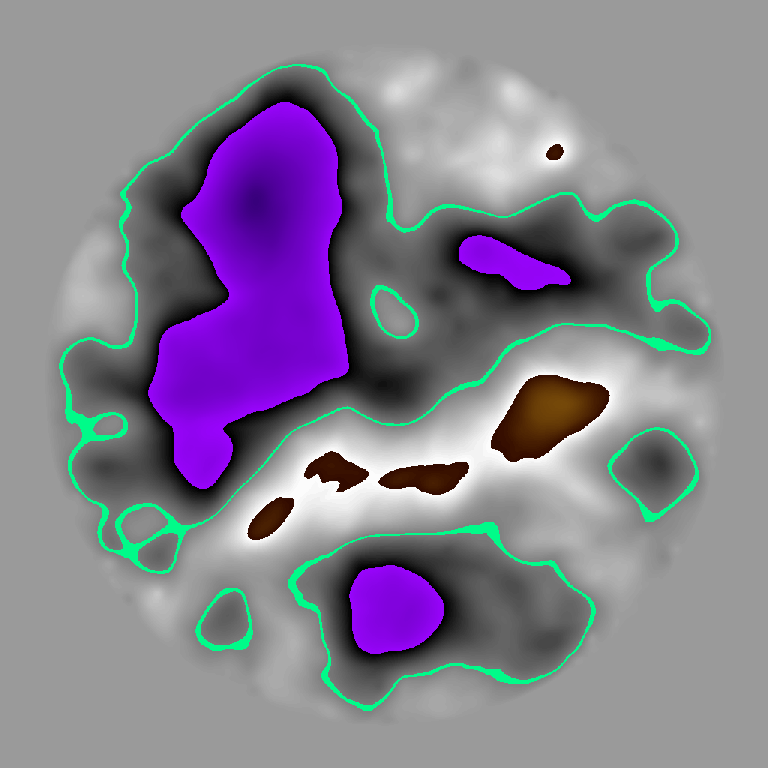Total streamfunction (min=-1.18 / max=0.60)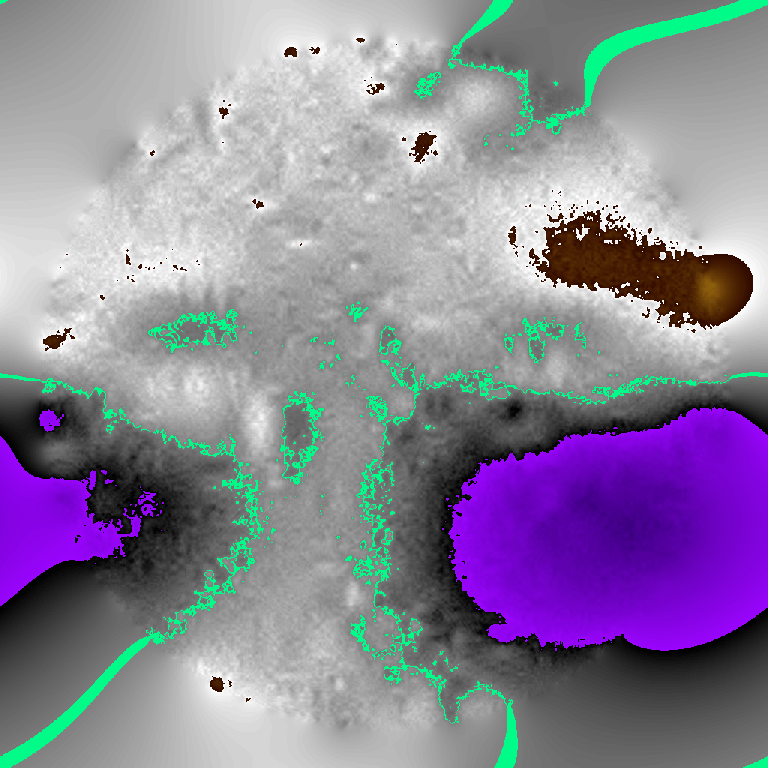Figure: Coherent streamfunction (min=-1.18 / max= 0.60) and
incoherent streamfunction (min=-3E-03 / max= 2E-03)Vorticity versus streamfunction for the total flowVorticity versus streamfunction for the coherent flow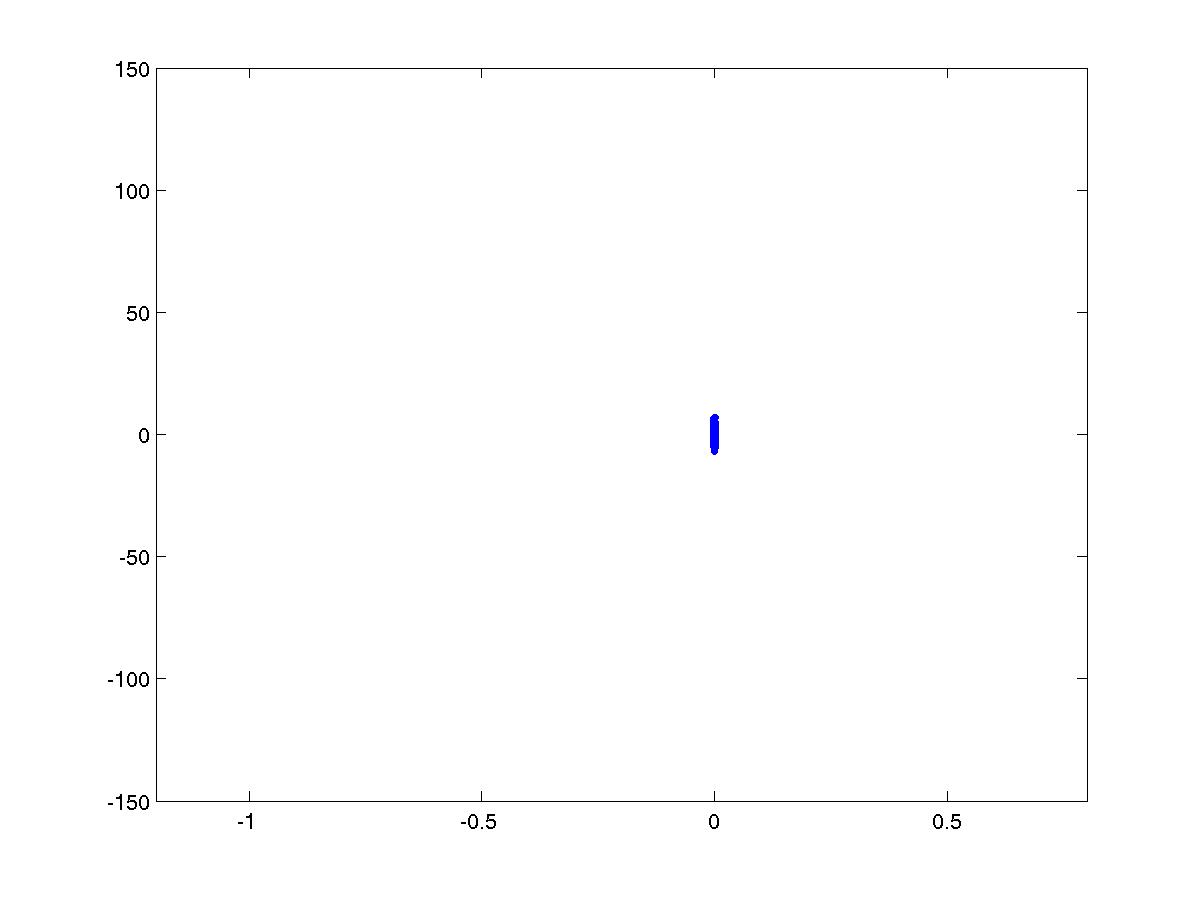Vorticity versus streamfunction for the incoherent flow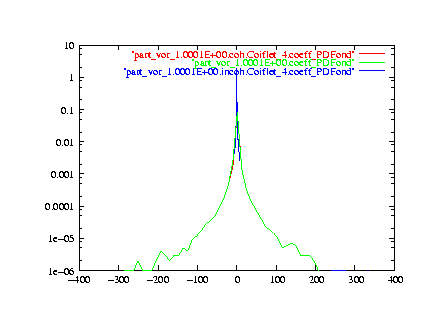Probability density function (PDF) of the wavelet coefficients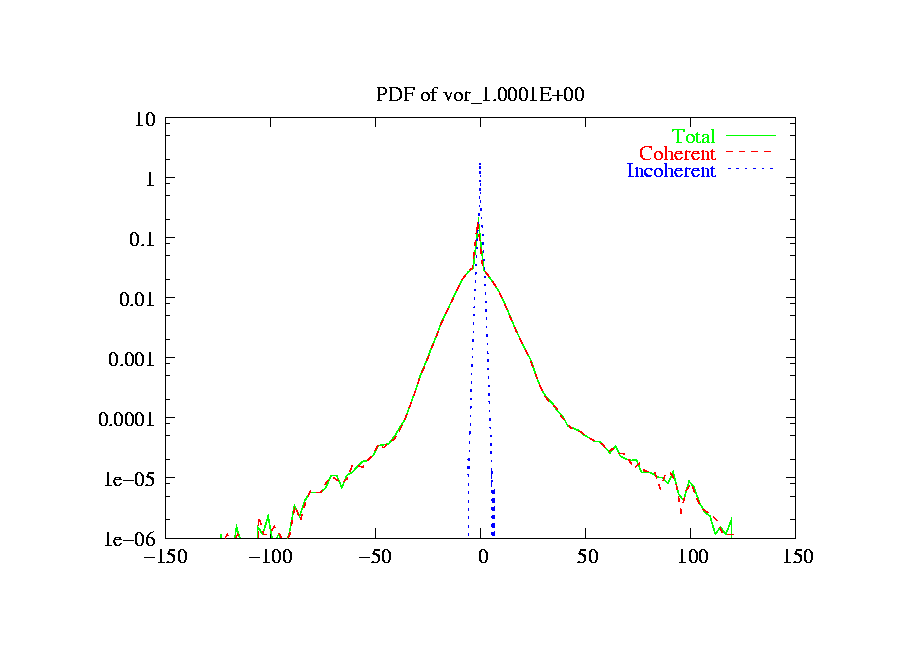Probability density function (PDF) of the vorticityFigure: Enstrophy spectrum in lin-lin coordinates
(total: green, coherent: red, incoherent: blue)Figure: Enstrophy spectra in log-log coordinates
(total: green, coherent: red, incoherent: blue)

### 2D COMPRESSIBLE FLOW: DNS OF SAINT-VENANT EQUATIONS

This experiment has been done using a pseudo-spectral code integrating the two-dimensional Saint-Venant equations in the Shallow-wather model without forcing. No-slip boundary conditions have been imposed in the cylindrical domain using volume penalisation.
The resolution is N=2562.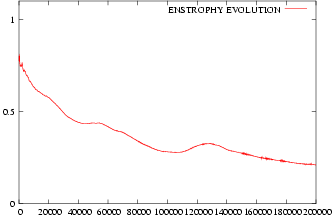Time evolution of the total enstophy z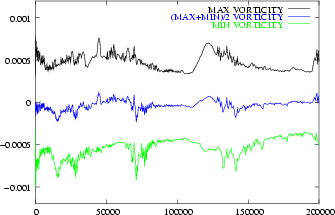Time evolution of the maximal, minimal and mean vorticityTime evolution of the total energy E

We split the total flow into coherent and incoherent flows using the CVS filtering method with the Coifman 12 wavelet.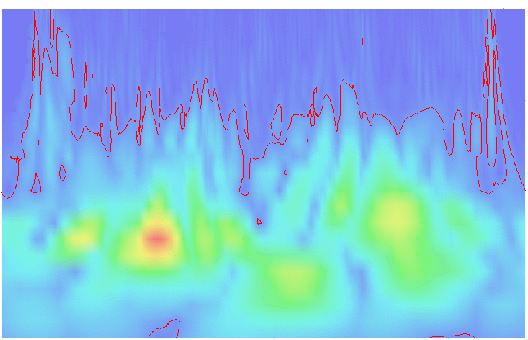Cut of the wavelet space with the treshold (red) separating coherent and incoherent wavelet modes

The percentage of retained coefficient is 2.8% N.
The percentage of retained enstrophy is 81.4% Z.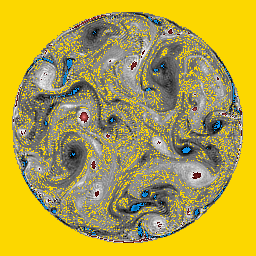Total vorticity (min=-123.63 / max=124.44)Coherent vorticity (min=-123 / max=122.9) and
incoherent vorticity (min=-6.78 / max=7.09)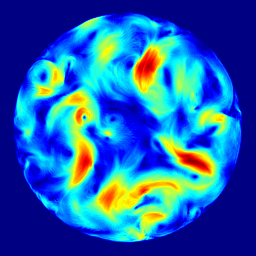Total velocity modulus (min=0 / max=2.67)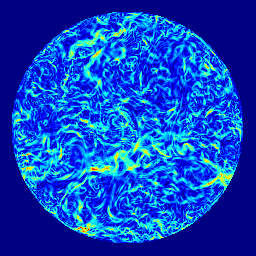Figure: Coherent velocity modulus (min=0 / max=2.67) and
incoherent velocity modulus (min=0 / max=0.03)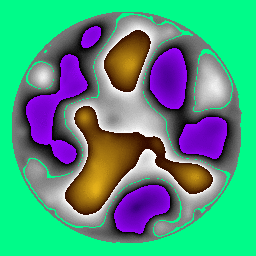Total streamfunction (min=-1.18 / max=0.60)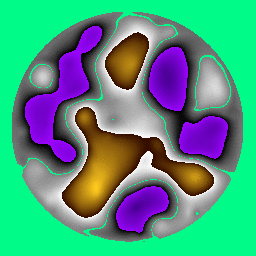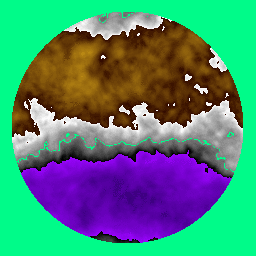Figure: Coherent streamfunction (min=-1.18 / max= 0.60) and
incoherent streamfunction (min=-3E-03 / max= 2E-03)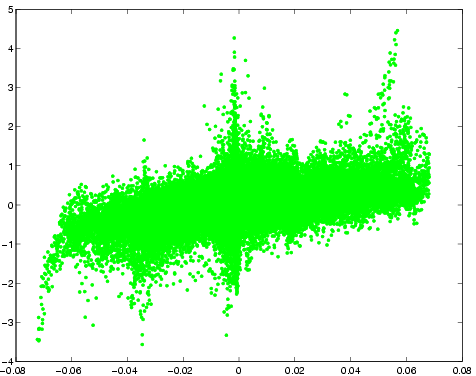Vorticity versus streamfunction for the total flowVorticity versus streamfunction for the coherent flow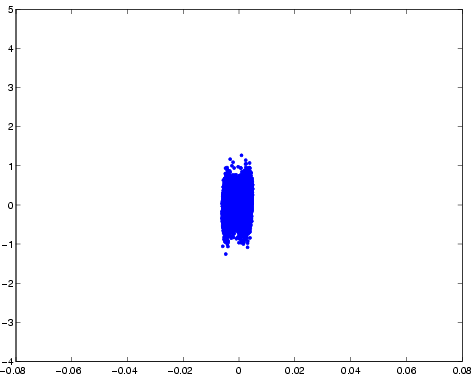Vorticity versus streamfunction for the incoherent flow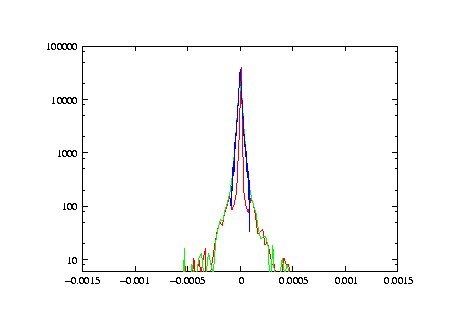Probability density function (PDF) of the wavelet coefficientsProbability density function (PDF) of the vorticity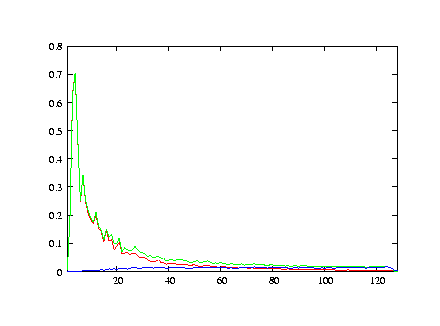Figure: Enstrophy spectrum in lin-lin coordinates
(total: green, coherent: red, incoherent: blue)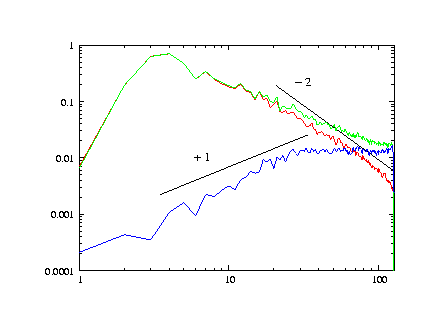Figure: Enstrophy spectra in log-log coordinates
(total: green, coherent: red, incoherent: blue)

### Perspectives

We plan to perform other numerical experiments:

• using a 2D Navier-Stokes code with forcing
• using a shallow water code with forcing.